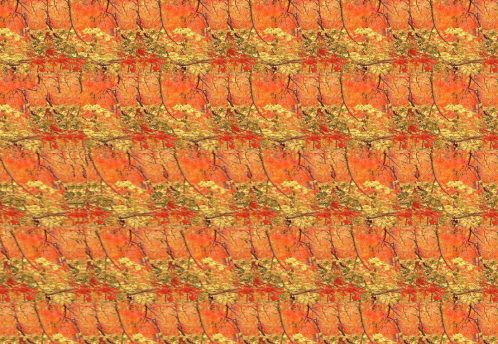# AutostereogramsOpening the images in full resolution may improve the effect
###### Autostereograms, 2004.

Note: if you are new to autostereograms, you can enter “how to view stereograms” in your search engine of choice to get a plenty of different approaches on how to see the 3D effect, to find which best suits you. In short – the goal is a focused double vision so that each eye sees it’s own fragment of repeating image, but they become combined by the brain. Can be easier to start by looking through the image up close and slowly pulling away while trying to look at a point a bit further away than the actual image is.

Being interested in 3D and inherently in perception of depth, stereograms caught my eye. In particular – repetition based autostereograms (stereo images), as the one having technologically simplest and closest to self-sufficient end product, while being the most synthetic. Meaning – being able to provide somewhat pure, detached perception of depth. This tipe of autostereograms is also known under brand name “Magic Eye”.

After a bit of experimentation I found that the principle behind repetition autostereorgams can be quite simple and created a rudimentary program to produce them. The main mechanism behind is to produce a horizontal pixel shift based on an arbitrary depth map (“Z-buffer”) in a repetitive pattern, factoring offset amount with depth value and pattern repetition width.

Main function source code:

``````[+] Expand[-] Collapse/*
Note: I was self-teaching programming at the time, hence the dreadful form. The source is mostly provided as a future note to myself :) Might replace with a proper version if I get back to stereograms.
*/
double emi,dem,per,dr,dg,db,x1,x2;
unsigned __int8 cr,cg,cb,fr,fg,fb;
emi=(double)255/100*CSpinEdit2->Value;//Depth -% of 255, UI?

for(int z=(Image1->Width/CSpinEdit1->Value)-1; z>=0; z--){//CSpinEdit1: Pereod size
//iterate for every pattern repetition?
for(int x=Image1->Width; x>0; x--){
for(int y=0; y<Image1->Height; y++){
//get depth
dem=(double)GetRValue(Image2->Canvas->Pixels[x][y])/100*CSpinEdit2->Value;//depth magnitude
for(per=dem; per>=1; per--){}//I didn't know how to modulo???

x1=x-(z*CSpinEdit1->Value)-(dem-per);
x2=x-(z*CSpinEdit1->Value)-(dem-per+1);
if(x1<0)
{cr=GetRValue(Image1->Canvas->Pixels[x1+Image1->Width][y]);
cg=GetGValue(Image1->Canvas->Pixels[x1+Image1->Width][y]);
cb=GetBValue(Image1->Canvas->Pixels[x1+Image1->Width][y]);}
else
{cr=GetRValue(Image1->Canvas->Pixels[x1][y]);
cg=GetGValue(Image1->Canvas->Pixels[x1][y]);
cb=GetBValue(Image1->Canvas->Pixels[x1][y]);}
if(x2<0)
{fr=GetRValue(Image1->Canvas->Pixels[x2+Image1->Width][y]);
fg=GetGValue(Image1->Canvas->Pixels[x2+Image1->Width][y]);
fb=GetBValue(Image1->Canvas->Pixels[x2+Image1->Width][y]);}
else
{fr=GetRValue(Image1->Canvas->Pixels[x2][y]);
fg=GetGValue(Image1->Canvas->Pixels[x2][y]);
fb=GetBValue(Image1->Canvas->Pixels[x2][y]);}
if(per!=0)dr=(fr*per)+(cr*(1-per));
else dr=cr;
if(per!=0)dg=(fg*per)+(cg*(1-per));
else dg=cg;
if(per!=0)db=(fb*per)+(cb*(1-per));
else db=cb;
Image1->Canvas->Pixels[x-(z*CSpinEdit1->Value)][y]=RGB(dr,dg,db);
}}Image1->Refresh();}
ShowMessage("Complete!");``````

Depth maps: